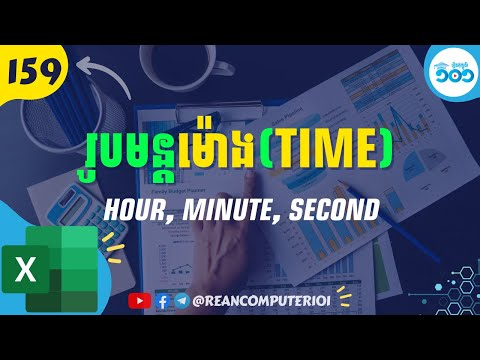## How to use HOUR, MINUTE, SECOND and TIME in Excel with Example Download

If you have date time value in excel and want to get separate value of them - HOUR, MINUTE and SECOND function get you cover. And if you have separate value of HOUR, MINUTE and SECOND, then you want to group together then TIME formula is used.### HOUR formula in Excel

HOUR returns value of hour from date-time as number from 0 (12:00 A.M.) to 23 (11:00 P.M.).
FORMULA:
=HOUR(serial_number)

◾ Serial_number: Required. Basically is time or date with time.

### MINUTE formula in Excel

MINUTE returns minute from date-time or time as integer from 0 to 59.
FORMULA:
=MINUTE(serial_number)

◾ Serial_number: Required. Basically is time or date with time.

### SECOND formula in Excel

SECOND returns value as second from date-time or time value as integer which range from 0 to 59.
FORMULA:
=SECOND(serial_number)

◾ Serial_number: Required. Basically is time or date with time.

### TIME formula in Excel

TIME is used to put together of hour, minute and second to become time format.
FORMULA:
=TIME(hour,minute,second)

◾ hour Required. The value of hour.
◾ minute Required. The value of minute.
◾ second Required. The value of second.

Watch How to use HOUR, MINUTE, SECOND and TIME in Excel speak Khmer